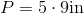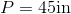# ISEE Upper Level Math : How to find the perimeter of a pentagon

## Example Questions

### Example Question #1 : How To Find The Perimeter Of A Pentagon

Find the perimeter of a pentagon with a side of length 12in.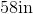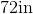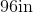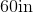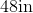Explanation:

To find the perimeter of a pentagon, we will use the following formula: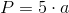where a is the length of one side of the pentagon.

Now, we know the length of one side of the pentagon is 12in.

Knowing this, we can substitute into the formula.  We get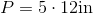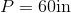### Example Question #2 : How To Find The Perimeter Of A Pentagon

Find the perimeter of a pentagon with a side having a length of 21in.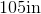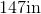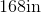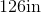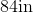Explanation:

A pentagon has 5 sides.  To find the perimeter, we will use the following formula: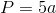where a is the length of a side of the pentagon.

Now, we know the length of a side of the pentagon is 21in.

Knowing this, we will substitute into the formula. We get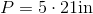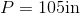### Example Question #3 : How To Find The Perimeter Of A Pentagon

Find the perimeter of a pentagon with a side having a length of 15cm.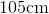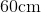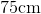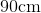Explanation:

To find the perimeter of a pentagon, we will use the following formula:where a is the length of one side of the pentagon.  Because a pentagon has 5 equal sides, we can use any side in the formula.

So, we know the length of one side of the pentagon is 15cm.  So, we can substitute.  We get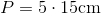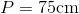### Example Question #4 : How To Find The Perimeter Of A Pentagon

A pentagon has a side of length 11in.  Find the perimeter.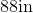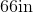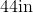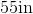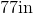Explanation:

To find the perimeter of a pentagon, we will use the following formula:where a is the length of one side of the pentagon.

Now, we know the length of one side of the pentagon is 11in.

Knowing this, we can substitute into the formula.  We get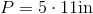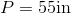### Example Question #5 : How To Find The Perimeter Of A Pentagon

Find the perimeter of a pentagon with a side having a length of 9in.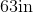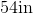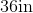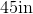Explanation:

A pentagon has 5 equal sides.  To find the perimeter of a pentagon, we will use the following formula:where a is the length of one side of the pentagon.

Now, we know a side of the pentagon has a length of 9in. So, we get